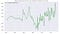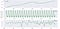# What’s The Difference Between Autocorrelation & Partial Autocorrelation For Time Series Analysis?

Two of the most important components to analyzing and forecasting with Time Series data are plotting — and reviewing— the Autocorrelation and Partial Autocorrelation functions.

Understanding the difference between these two calculations is the focus of this post, with the goal being to provide solid answers to the following:

• What’s Autocorrelation?

OK, let’s dive in.

First…

# A Quick Word On The General Purpose Of Correlation In Data Analysis

It’s useful to mention here that statistical correlation in general helps us to identify the nature of the relationships between variables, and that this is where ACF and PACF come in with respect to Time Series data.

Correlation can be positive, negative or even neutral between variables.

Knowing the nature of correlation between variables with a dataset— or time series observations— can help us understand whether we need to further prepare our data, or which model is best for machine learning.

OK, now onto some definitions:

# What’s Autocorrelation?

Autocorrelation is a calculation of the correlation of the time series observations with values of the same series, but at previous times.

The time steps at those previous times are referred to as lags.

# What’s Partial Autocorrelation?

Partial Autocorrelation, on the other hand, summarizes the relationship between an observation in a time series with observations at previous time steps, but with the relationships of intervening observations removed.

Essentially, the indirect correlations are removed.

This is the critical difference between Autocorrelation and Partial Autocorrelation — the inclusion or exclusion of indirect correlations in the calculation.

# Examples: an ACF and PACF of time series for Real Estate Housing prices

One thing to remember and mention here:

The one thing best to do before plotting an ACF or PACF with Time Series data is to identify stationarity for a time series.

This means that the Time Series is free of any trends or seasonality.

Actually, the ACF and PACF plots can help us to verify that the Time Series is stationary (as well as to ascertain the needed terms for modeling).

With my Time Series analysis & forecast of a time series derived from the well-known Zillow Housing dataset, I chose to focus on the Charlotte-and-surrounding areas.

After doing some feature engineering to determine Historical ROI and sorting the Series, I chose to first focus on zip code 28204.

Next, I performed a Dickey-Fuller test (to determine whether the Series was stationary) and found that it was not stationary in fact.

I then decided to try differencing the data, but even with this I was unable to achieve stationarity.Issues with covariance and homoscedasticity suggest a lack of stationarity as well.

The question then was:

What should I do?

Time Series models assume that the data modeled on will have stationarity.

I then chose to use the seasonal_decompose method to separate the Series into its trend, seasonality and residual components.

By de-trending and de-seasonalizing the Time Series, leaving only the residuals present in the Series, I was able to evaluate the residuals and confirm stationarity for these.

I also doubly confirmed by running a Dickey-Fuller test.Seasonal decomposition removes seasonality and trend, leaving only the residuals of the series.

So, the ACF and PACF plots helps us to confirm stationarity, but also as mentioned (and perhaps more importantly), we would use these to determine the proper non-seasonal and/or seasonal terms to use for modeling.

Now, let’s interpret an ACF and PCF plot.

# Interpreting an ACF plot

The below graphic shows both ACF and PACF plots of the residuals from the above time series on which seasonal decomposition was applied.

With the below ACF plot, notice the lags total to 50.

As well, we can see that the correlation scale is from -1 to 1, which represents a scale from zero correlation to full correlation.

With there being more than 20 lags with a positive correlation above 0.5 there’s a good chance this time series will need a significant level of differencing to achieve stationarity.

We remember:

Autocorrelation is a calculation of the correlation of the time series observations with values of the same series, but at previous times.

But also, we remember:

Partial Autocorrelation, on the other hand, summarizes the relationship between an observation in a time series with observations at previous time steps, but with the relationships of intervening observations removed.

Essentially, the indirect correlations are removed.

So, in the PACF plot above, we can see this happening. Because the indirect correlations are not calculated, the correlation “legs” dwindle completely to zero. There is much more to these calculations, of course, but a high-level understanding of PACF is rooted in its effort to remove indirect correlations.

Moreover, these two plots can help us decide on the correct non-seasonal and seasonal terms to use for our modeling.

For example, the PACF shows us — because two positive partial autocorrelations are present — that the higher-order autocorrelations are essentially explained by the lag-2 autocorrelation.

With inspection of the PACF, we can learn how many AR terms we need to use to explain the autocorrelation pattern in a time series.

If the Partial Autocorrelation is significant at only these two lags and the remaining lags abruptly fall to zero, it suggests using an AR term of 2.

AR stands for Autoregressive.

This is a basic breakdown example of what interpreting ACF and PACF plots for time series consists of.

Overall, both Autocorrelation and Partial Autocorrelation are fundamental calculations to know when analyzing and forecasting with time series data.

Copywriter & Website specialist | practicing ma’at & meditation | Student of data science & decentralization

## More from Marvin Lee

Copywriter & Website specialist | practicing ma’at & meditation | Student of data science & decentralization